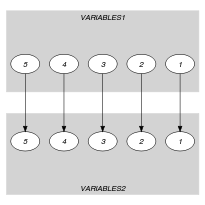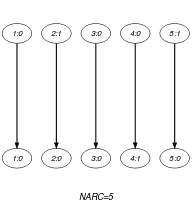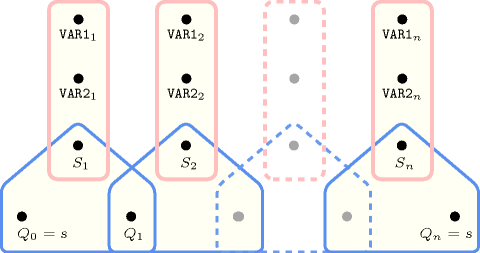## 5.32. arith_or

Origin

Used in the definition of several automata

Constraint

$\mathrm{𝚊𝚛𝚒𝚝𝚑}_\mathrm{𝚘𝚛}\left(\mathrm{𝚅𝙰𝚁𝙸𝙰𝙱𝙻𝙴𝚂}\mathtt{1},\mathrm{𝚅𝙰𝚁𝙸𝙰𝙱𝙻𝙴𝚂}\mathtt{2},\mathrm{𝚁𝙴𝙻𝙾𝙿},\mathrm{𝚅𝙰𝙻𝚄𝙴}\right)$

Arguments
 $\mathrm{𝚅𝙰𝚁𝙸𝙰𝙱𝙻𝙴𝚂}\mathtt{1}$ $\mathrm{𝚌𝚘𝚕𝚕𝚎𝚌𝚝𝚒𝚘𝚗}\left(\mathrm{𝚟𝚊𝚛}-\mathrm{𝚍𝚟𝚊𝚛}\right)$ $\mathrm{𝚅𝙰𝚁𝙸𝙰𝙱𝙻𝙴𝚂}\mathtt{2}$ $\mathrm{𝚌𝚘𝚕𝚕𝚎𝚌𝚝𝚒𝚘𝚗}\left(\mathrm{𝚟𝚊𝚛}-\mathrm{𝚍𝚟𝚊𝚛}\right)$ $\mathrm{𝚁𝙴𝙻𝙾𝙿}$ $\mathrm{𝚊𝚝𝚘𝚖}$ $\mathrm{𝚅𝙰𝙻𝚄𝙴}$ $\mathrm{𝚒𝚗𝚝}$
Restrictions
 $\mathrm{𝚛𝚎𝚚𝚞𝚒𝚛𝚎𝚍}$$\left(\mathrm{𝚅𝙰𝚁𝙸𝙰𝙱𝙻𝙴𝚂}\mathtt{1},\mathrm{𝚟𝚊𝚛}\right)$ $\mathrm{𝚛𝚎𝚚𝚞𝚒𝚛𝚎𝚍}$$\left(\mathrm{𝚅𝙰𝚁𝙸𝙰𝙱𝙻𝙴𝚂}\mathtt{2},\mathrm{𝚟𝚊𝚛}\right)$ $|\mathrm{𝚅𝙰𝚁𝙸𝙰𝙱𝙻𝙴𝚂}\mathtt{1}|=|\mathrm{𝚅𝙰𝚁𝙸𝙰𝙱𝙻𝙴𝚂}\mathtt{2}|$ $\mathrm{𝚁𝙴𝙻𝙾𝙿}\in \left[=,\ne ,<,\ge ,>,\le \right]$
Purpose

Enforce for all pairs of variables $\mathrm{𝚟𝚊𝚛}{\mathtt{1}}_{i},\mathrm{𝚟𝚊𝚛}{\mathtt{2}}_{i}$ of the $\mathrm{𝚅𝙰𝚁𝙸𝙰𝙱𝙻𝙴𝚂}\mathtt{1}$ and $\mathrm{𝚅𝙰𝚁𝙸𝙰𝙱𝙻𝙴𝚂}\mathtt{2}$ collections to have $\mathrm{𝚟𝚊𝚛}{\mathtt{1}}_{i}\mathrm{𝚁𝙴𝙻𝙾𝙿}\mathrm{𝚅𝙰𝙻𝚄𝙴}\vee \mathrm{𝚟𝚊𝚛}{\mathtt{2}}_{i}\mathrm{𝚁𝙴𝙻𝙾𝙿}\mathrm{𝚅𝙰𝙻𝚄𝙴}$.

Example
$\left(〈0,1,0,0,1〉,〈0,0,0,1,0〉,=,0\right)$

The constraint $\mathrm{𝚊𝚛𝚒𝚝𝚑}_\mathrm{𝚘𝚛}$ holds since, for all pairs of variables $\mathrm{𝚟𝚊𝚛}{\mathtt{1}}_{i},\mathrm{𝚟𝚊𝚛}{\mathtt{2}}_{i}$ of the $\mathrm{𝚅𝙰𝚁𝙸𝙰𝙱𝙻𝙴𝚂}\mathtt{1}$ and $\mathrm{𝚅𝙰𝚁𝙸𝙰𝙱𝙻𝙴𝚂}\mathtt{2}$ collections, there is at least one variable that is equal to 0.

Typical
 $|\mathrm{𝚅𝙰𝚁𝙸𝙰𝙱𝙻𝙴𝚂}\mathtt{1}|>0$ $\mathrm{𝚁𝙴𝙻𝙾𝙿}\in \left[=\right]$
Symmetries
• Arguments are permutable w.r.t. permutation $\left(\mathrm{𝚅𝙰𝚁𝙸𝙰𝙱𝙻𝙴𝚂}\mathtt{1},\mathrm{𝚅𝙰𝚁𝙸𝙰𝙱𝙻𝙴𝚂}\mathtt{2}\right)$ $\left(\mathrm{𝚁𝙴𝙻𝙾𝙿}\right)$ $\left(\mathrm{𝚅𝙰𝙻𝚄𝙴}\right)$.

• Items of $\mathrm{𝚅𝙰𝚁𝙸𝙰𝙱𝙻𝙴𝚂}\mathtt{1}$ and $\mathrm{𝚅𝙰𝚁𝙸𝙰𝙱𝙻𝙴𝚂}\mathtt{2}$ are permutable (same permutation used).

Arg. properties

Contractible wrt. $\mathrm{𝚅𝙰𝚁𝙸𝙰𝙱𝙻𝙴𝚂}\mathtt{1}$ and $\mathrm{𝚅𝙰𝚁𝙸𝙰𝙱𝙻𝙴𝚂}\mathtt{2}$ (remove items from same position).

specialisation: $\mathrm{𝚊𝚛𝚒𝚝𝚑}$ ($\mathrm{𝚟𝚊𝚛𝚒𝚊𝚋𝚕𝚎}$ $\mathrm{𝚁𝙴𝙻𝙾𝙿}$ $\mathrm{𝚅𝙰𝙻𝚄𝙴}$ $\vee$ $\mathrm{𝚟𝚊𝚛𝚒𝚊𝚋𝚕𝚎}$ $\mathrm{𝚁𝙴𝙻𝙾𝙿}$ $\mathrm{𝚅𝙰𝙻𝚄𝙴}$ replaced by $\mathrm{𝚟𝚊𝚛𝚒𝚊𝚋𝚕𝚎}$ $\mathrm{𝚁𝙴𝙻𝙾𝙿}$ $\mathrm{𝚅𝙰𝙻𝚄𝙴}$).

Keywords
Arc input(s)

$\mathrm{𝚅𝙰𝚁𝙸𝙰𝙱𝙻𝙴𝚂}\mathtt{1}$ $\mathrm{𝚅𝙰𝚁𝙸𝙰𝙱𝙻𝙴𝚂}\mathtt{2}$

Arc generator
$\mathrm{𝑃𝑅𝑂𝐷𝑈𝐶𝑇}$$\left(=\right)↦\mathrm{𝚌𝚘𝚕𝚕𝚎𝚌𝚝𝚒𝚘𝚗}\left(\mathrm{𝚟𝚊𝚛𝚒𝚊𝚋𝚕𝚎𝚜}\mathtt{1},\mathrm{𝚟𝚊𝚛𝚒𝚊𝚋𝚕𝚎𝚜}\mathtt{2}\right)$

Arc arity
Arc constraint(s)
$\mathrm{𝚟𝚊𝚛𝚒𝚊𝚋𝚕𝚎𝚜}\mathtt{1}.\mathrm{𝚟𝚊𝚛}\mathrm{𝚁𝙴𝙻𝙾𝙿}\mathrm{𝚅𝙰𝙻𝚄𝙴}\vee \mathrm{𝚟𝚊𝚛𝚒𝚊𝚋𝚕𝚎𝚜}\mathtt{2}.\mathrm{𝚟𝚊𝚛}\mathrm{𝚁𝙴𝙻𝙾𝙿}\mathrm{𝚅𝙰𝙻𝚄𝙴}$
Graph property(ies)
$\mathrm{𝐍𝐀𝐑𝐂}$$=|\mathrm{𝚅𝙰𝚁𝙸𝙰𝙱𝙻𝙴𝚂}\mathtt{1}|$

Graph class
 $•$$\mathrm{𝙰𝙲𝚈𝙲𝙻𝙸𝙲}$ $•$$\mathrm{𝙱𝙸𝙿𝙰𝚁𝚃𝙸𝚃𝙴}$ $•$$\mathrm{𝙽𝙾}_\mathrm{𝙻𝙾𝙾𝙿}$

Graph model

Parts (A) and (B) of Figure 5.32.1 respectively show the initial and final graphs associated with the Example slot. Since we use the $\mathrm{𝐍𝐀𝐑𝐂}$ graph property, the arcs of the final graph are stressed in bold.

##### Figure 5.32.1. Initial and final graph of the $\mathrm{𝚊𝚛𝚒𝚝𝚑}_\mathrm{𝚘𝚛}$ constraint(a) (b)
Automaton

Figure 5.32.2 depicts the automaton associated with the $\mathrm{𝚊𝚛𝚒𝚝𝚑}_\mathrm{𝚘𝚛}$ constraint. Let $\mathrm{𝚅𝙰𝚁}{\mathtt{1}}_{i}$ and $\mathrm{𝚅𝙰𝚁}{\mathtt{2}}_{i}$ be the ${i}^{th}$ variables of the $\mathrm{𝚅𝙰𝚁𝙸𝙰𝙱𝙻𝙴𝚂}\mathtt{1}$ and $\mathrm{𝚅𝙰𝚁𝙸𝙰𝙱𝙻𝙴𝚂}\mathtt{2}$ collections. To each pair of variables $\left(\mathrm{𝚅𝙰𝚁}{\mathtt{1}}_{i},\mathrm{𝚅𝙰𝚁}{\mathtt{2}}_{i}\right)$ corresponds a signature variable ${S}_{i}$. The following signature constraint links $\mathrm{𝚅𝙰𝚁}{\mathtt{1}}_{i}$, $\mathrm{𝚅𝙰𝚁}{\mathtt{2}}_{i}$ and ${S}_{i}$: $\mathrm{𝚅𝙰𝚁}{\mathtt{1}}_{i}\mathrm{𝚁𝙴𝙻𝙾𝙿}\mathrm{𝚅𝙰𝙻𝚄𝙴}\vee \mathrm{𝚅𝙰𝚁}{\mathtt{2}}_{i}\mathrm{𝚁𝙴𝙻𝙾𝙿}\mathrm{𝚅𝙰𝙻𝚄𝙴}⇔{S}_{i}$. The automaton enforces for each pair of variables $\mathrm{𝚅𝙰𝚁}{\mathtt{1}}_{i}$,$\mathrm{𝚅𝙰𝚁}{\mathtt{2}}_{i}$ the condition $\mathrm{𝚅𝙰𝚁}{\mathtt{1}}_{i}\mathrm{𝚁𝙴𝙻𝙾𝙿}\mathrm{𝚅𝙰𝙻𝚄𝙴}\vee \mathrm{𝚅𝙰𝚁}{\mathtt{2}}_{i}\mathrm{𝚁𝙴𝙻𝙾𝙿}\mathrm{𝚅𝙰𝙻𝚄𝙴}$.

##### Figure 5.32.2. Automaton of the $\mathrm{𝚊𝚛𝚒𝚝𝚑}_\mathrm{𝚘𝚛}$ constraint##### Figure 5.32.3. Hypergraph of the reformulation corresponding to the automaton of the $\mathrm{𝚊𝚛𝚒𝚝𝚑}_\mathrm{𝚘𝚛}$ constraint